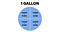# How Many Quarts Are in a Gallon

There are 4 quarts in 1 gallon.

This is easy to remember. Consider the word “quart”. It refers to a quarter.

When speaking about volumes, a quart is a quarter of a gallon. In other words, it is one-fourth of a gallon—so there are four of them in a gallon.This is an easy way to remember how many quarts are in a gallon

# How to Convert Between Gallons and Quarts

You can use a simple formula to figure out the number of quarts in gallons:

`quarts = 4 * gallons                                             (1)`

And to get the number of gallons given quarts, you can invert this formula:

`gallons = 0.25 * quarts                                          (2)`

## Examples

Example 1. How many quarts are in 1.5 gallons?

Answer. Let’s plug the numbers into formula 1:

`quarts = 4 * 1.5 = 6.0`

There are 6 quarts in 1.5 gallons

Example 2. How many gallons are 12 quarts?

Answer. Let’s plug the numbers into formula 2:

`gallons = 0.25 * 12 = 3.0`

12 quarts means 3 gallons.